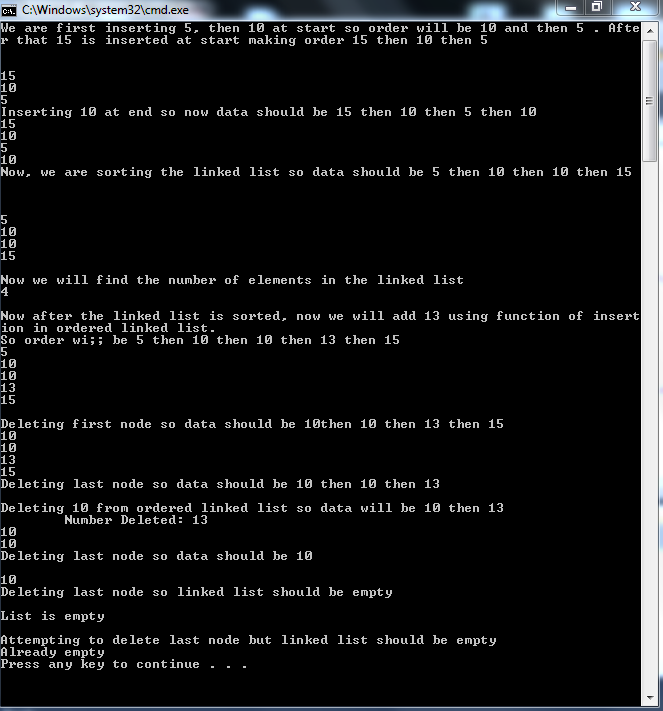# Linked List using C++ part5

`void insert_order (int val)	{		if(checkempty())		{			InsertAtStart(val);			return;		}		Node *new_node;		new_node= new Node;		new_node->data=val;		Node *prev, *cur;		prev=NULL;		cur=head;		if(val<head->data)		{			new_node->next=head;			head=new_node;			cout<<"\tNumber Inserted: "<<val<<endl;			return;		}		else 			{while(cur!=NULL && val>cur->data)		{			prev=cur;			cur=cur->next;		}		new_node->next=cur;		prev->next=new_node;}}`
`void delete_order(int val)	{		Node *cur;		cur=head;		if (checkempty())		{			cout<<"Already empty"<<endl;		}		else if(head->data==val)		{			head=head->next;			delete cur;			cur=NULL;			return;		}		else {while(cur->next!=NULL)		{			if(cur->next->data==val)			{				Node *d;				d=cur->next;				cur->next=cur->next->next;				cout<<"\tNumber Deleted: "<<val<<endl;				delete d;				return;			}			cur=cur->next;		}		}		cout<<" Number not found in the LIST\n"; }`
Given above is the code for deleting a node in linked list . First you check whether the linked list is empty or not. If it is then deletion is not possible and if first element is to be deleted then We simply make a pointer and make it point to first element and then we make head point to second node and then deleting first node. If this is not the first element then we simply make a pointer and make it traverse till the node we want to remove and simply change to next pointer of node which is behind the node we want to delete to the node next to the node we want to delete and simply delete the node we want to delete. Note : In next tutorial , copy constructor which has been deliberately delayed so that you would understand the basic concepts will be discussed and other than the copy constructor other functions would also be discussed . Output: